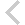FICO Xpress Optimization Examples Repository
 FICO Optimization Community FICO Xpress Optimization HomeApply a binary fixing heuristic to an unpresolved MIP problem

Description

We take a production plan model and solve its LP relaxation.

Next we fix those binary variables that are very near zero to 0.0, and those that are almost one to 1.0, by changing their respective upper and lower bounds. Finally, we solve the modified problem as a MIP.

This heuristic will speed up solution - though may fail to optimse the problem.

The results are displayed on screen and the problem statistics stored in a log file.

Source Files
By clicking on a file name, a preview is opened at the bottom of this page.

Data Files

FixBV.vb

Imports System
Imports Microsoft.VisualBasic
Imports Optimizer
Imports System.IO

Module FixBV
Private Const TOL As Double = 0.0005      ' Tolerance on binary variables

Public Sub RunFixBV(ByRef Log As TextWriter)
Try
Const sProblem As String = "coco"                 ' Problem name
Const sLogFile As String = "fixbv.log"            ' Log file name

Dim nCol As Integer                 ' Number of columns

' Global problem information
Dim nGlEnt As Integer               ' Number of global entities: binary, integer,
' semi-continuous and partial integer variables
Dim nSet As Integer                 ' Number of S1 and S2 sets
Dim pGlInd() As Integer             ' Column indices of the global entities
Dim pGlType() As Char               ' Global entity types

' Bound changes
Dim pBndInd() As Integer            ' Column indices of the bounds to be changed
Dim pBndType() As Char              ' New bound type
Dim pBndVal() As Double             ' New bound values
Dim nBnd As Integer                 ' Bound counter
Dim i As Integer                    ' Loop counter
Dim j As Integer                    ' Holder for the bound indices

' Solution information
Dim x() As Double                   ' LP solution values
Dim nGlStatus As Integer            ' Global status
Dim nNodes As Integer               ' Number of nodes solves so far in global search
Dim dObjVal As Double               ' Objective value of the best integer solution

' Initialise Optimizer
XPRS.Init("")

Dim prob As XPRSprob
prob = New XPRSprob

' Delete and define log file
If (File.Exists(sLogFile)) Then
File.Delete(sLogFile)
End If
prob.SetLogFile(sLogFile)

' Tell Optimizer to call HandleOptimizerMessage whenever a message is output

' Turn off presolve and permit no cuts - to slow down solution and allow
' the effect of the heuristic to be seen
prob.Presolve = 0
prob.CutStrategy = 0

' Solve the LP relaxation

' Get the number of columns
nCol = prob.Cols

' Allocate memory for solution array and check for memory shortage
ReDim x(nCol - 1)

' Solve the LP
prob.Maxim("")

' Get the LP solution values
prob.GetSol(x, Nothing, Nothing, Nothing)

' Fix the binary variables that are at their bounds

' Allocate memory for gloval entity arrays
ReDim pGlInd(nCol - 1)
ReDim pGlType(nCol - 1)

' Get global entity information
prob.GetGlobal(nGlEnt, nSet, pGlType, pGlInd, Nothing, Nothing, DirectCast(Nothing, Long()), Nothing, Nothing)

' Allocate memory for bound arrays
ReDim pBndInd(nGlEnt - 1)
ReDim pBndVal(nGlEnt - 1)
ReDim pBndType(nGlEnt - 1)

' Initialise the bound counter
nBnd = 0

' Go through the gloval entities
For i = 0 To nGlEnt - 1
' Test whether each is a binary variable
If (pGlType(i) = "B") Then
' Hold the index of the BV
j = pGlInd(i)

' If the value of the BV is within TOL of zero, store its index,
'   set its upper bound to 0, and increment the bound counter
If (x(j) <= TOL) Then
pBndInd(nBnd) = j
pBndType(nBnd) = "U"
pBndVal(nBnd) = 0.0
nBnd = nBnd + 1

' If the value of the BV is within TOL of one, store its index,
'   set its lower bound to 1, and increment the bound counter
ElseIf ((1 - x(j)) <= TOL) Then
pBndInd(nBnd) = j
pBndType(nBnd) = "L"
pBndVal(nBnd) = 1.0
nBnd = nBnd + 1
End If
End If
Next

' Instruct the Optimizer to change the bounds of the appropriate BVs,
'   and tell the user how many have been fixed
prob.ChgBounds(nBnd, pBndInd, pBndType, pBndVal)

Log.WriteLine("Solving problem {0} with a binary fixing heuristic" & _
vbCrLf & vbCrLf, sProblem)
Log.WriteLine("   After the LP optiziation {0} binary variables were fixed" & _
vbCrLf & vbCrLf, nBnd)

' Solve the modified problem as a MIP

' Search for an integer solution
prob.[Global]()

' Get th enumber of nodes solved in the global search
nNodes = prob.Nodes

' Get the objective value of the best integer solution
dObjVal = prob.MIPObjVal

' Check the global status and display the results of the global search
nGlStatus = prob.MIPStatus

Select Case nGlStatus
Case 0
Log.WriteLine("  Problem has not been loaded")
Case 1
Log.WriteLine("  Search has not begun - LP has not been optimised")
Case 2
Log.WriteLine("  Search has not begun - LP has been optimised")
Case 3
Log.WriteLine("  Search interrupted - No integer solution was found")
Case 4
Log.WriteLine("  Search interrupted - Integer solution found: %g", dObjVal)
Case 5
Log.WriteLine("  No integer solution was found")
Case 6
Log.WriteLine("  Integer solution found: {0}", dObjVal)
End Select
Log.WriteLine(vbCrLf & vbCrLf & "The MIP optimisation took {0} nodes" & vbCrLf & vbCrLf, nNodes)

' Destroy the problem and free the optimizer
prob.Destroy()
XPRS.Free()

Catch ex As Exception
Log.WriteLine(ex.ToString)
End Try
End Sub

Private Sub HandleOptimizerMessage(ByVal prob As Optimizer.XPRSprob, ByVal data As Object, _
ByVal message As String, ByVal len As Integer, _
ByVal msglvl As Integer)
Dim log As TextWriter
log = data
If (msglvl = 3 Or msglvl = 4) Then
log.WriteLine(message)
End If
End Sub

End Module